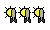Book 32 Mathematics 1959
 ◀ Words of Science The Living River [The Bloodstream] ▶Asimov fanTarget reader

The body’s transit system

The most important tool of science is mathematics. This clear and readable book shows even the non-mathematical reader how to  use this tool with understanding. Starting with the most basic sort of finger counting, Isaac Asimov proceeds to the pleasures of the abacus, where numbers take physical shapes, and on to the ideas of zero, fractions, and the decimal system. He makes sense of logarithms and even of imaginary numbers, and ends at the very frontiers of mathematics with a discussion of infinity and the concept of an infinity of infinities! He mathematics which Professor Asimov presents is not the thorny wasteland many struggling students suppose it to be. His main concern is no the mathematical techniques one learns in textbooks, but the various wherefores behind them.

This is the first of Asimov’s three “Realm” books published with Houghton-Mifflin (OK, four, if you count An Easy Introduction to the Slide Rule). This is a book that Asimov was very fond of, partly because it whipped if off in just a few weeks, and partly because he managed to go from counting on fingers to transfinite quantities in the space of fewer than 150 pages.

The book is rather breezy, and I’m not sure how well it would work as an introduction to the more basic subjects if someone doesn’t know about them (such as multiplication and division). On the other hand, the book does a marvelous job in explaining the why of our numeral system, the four basic arithmetic operations, powers, logarithms, complex numbers, and so on, and, in the later sections, the fact that most of us do not actually need to use complex numbers and so on in our daily life means that it doesn’t really matter if you don’t leave the book able to multiply (7 + 3i) and (5 - 8i) in your head. (The answer is 59 - 41i, by the way.)

There are a couple of points that provoke a wry smile, perhaps, nowadays: talking about a slide rule, for one, or Asimov’s lament that inventing a new “The Sign” symbol instead of using “i.” Mathematics, however, unlike other sciences, does not get obsolete very easily, and there is little, if anything, in the book which is actually out-dated. The result is a nicely fresh little volume providing an enjoyable introduction to numbers and basic mathematics.